Скачать презентацию Chapter Eighteen Equity Valuation Models INVESTMENTS BODIE

43931a7d8106e92848569fee5a3589c1.ppt

• Количество слайдов: 42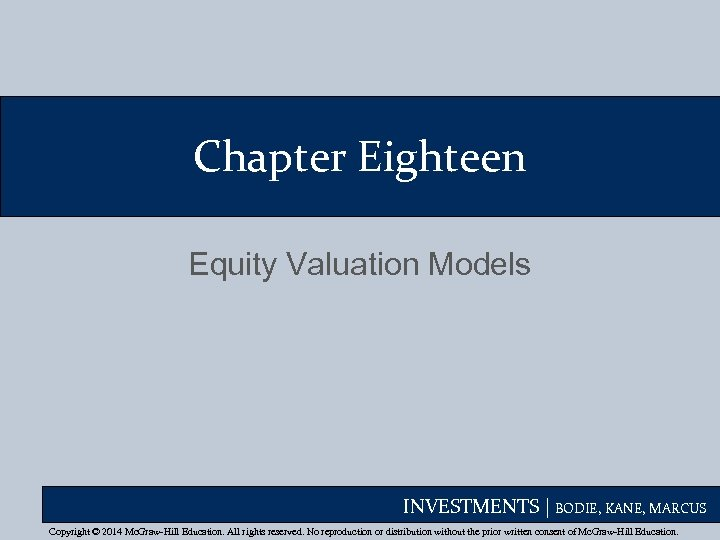Chapter Eighteen Equity Valuation Models INVESTMENTS | BODIE, KANE, MARCUS Copyright © 2014 Mc. Graw-Hill Education. All rights reserved. No reproduction or distribution without the prior written consent of Mc. Graw-Hill Education.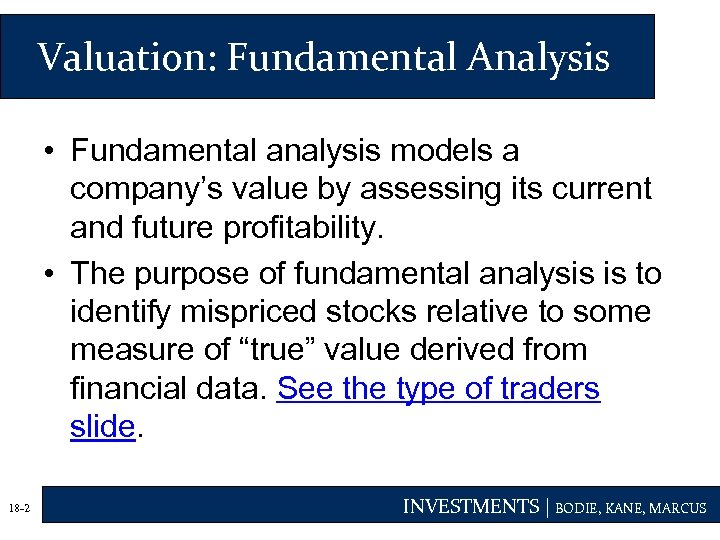Valuation: Fundamental Analysis • Fundamental analysis models a company’s value by assessing its current and future profitability. • The purpose of fundamental analysis is to identify mispriced stocks relative to some measure of “true” value derived from financial data. See the type of traders slide. 18 -2 INVESTMENTS | BODIE, KANE, MARCUS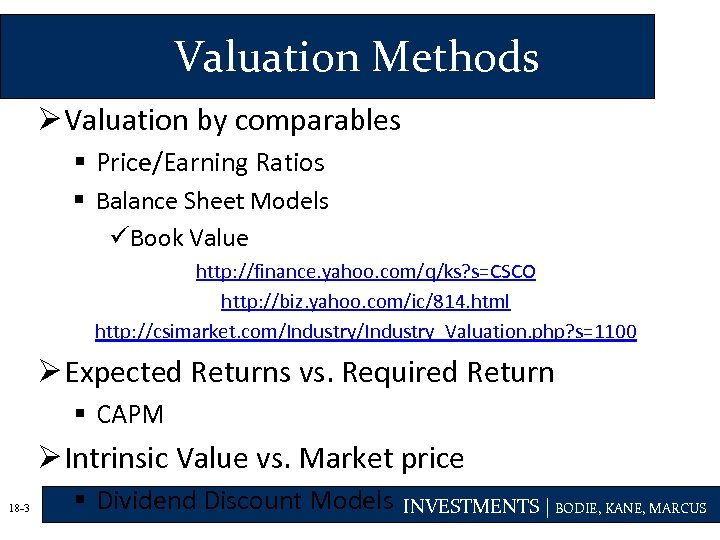Valuation Methods Ø Valuation by comparables § Price/Earning Ratios § Balance Sheet Models üBook Value http: //finance. yahoo. com/q/ks? s=CSCO http: //biz. yahoo. com/ic/814. html http: //csimarket. com/Industry_Valuation. php? s=1100 Ø Expected Returns vs. Required Return § CAPM Ø Intrinsic Value vs. Market price 18 -3 § Dividend Discount Models INVESTMENTS | BODIE, KANE, MARCUS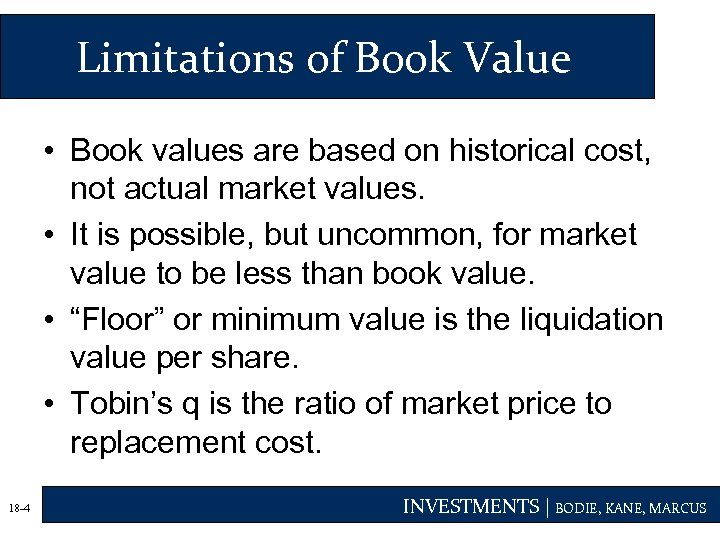Limitations of Book Value • Book values are based on historical cost, not actual market values. • It is possible, but uncommon, for market value to be less than book value. • “Floor” or minimum value is the liquidation value per share. • Tobin’s q is the ratio of market price to replacement cost. 18 -4 INVESTMENTS | BODIE, KANE, MARCUS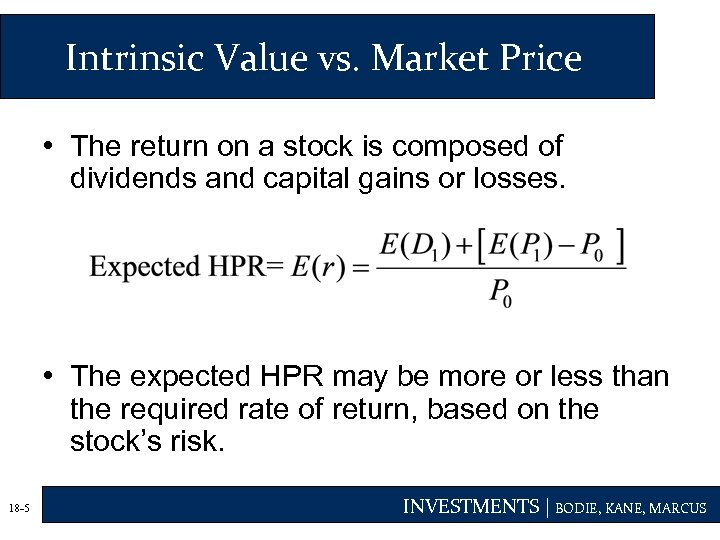Intrinsic Value vs. Market Price • The return on a stock is composed of dividends and capital gains or losses. • The expected HPR may be more or less than the required rate of return, based on the stock’s risk. 18 -5 INVESTMENTS | BODIE, KANE, MARCUS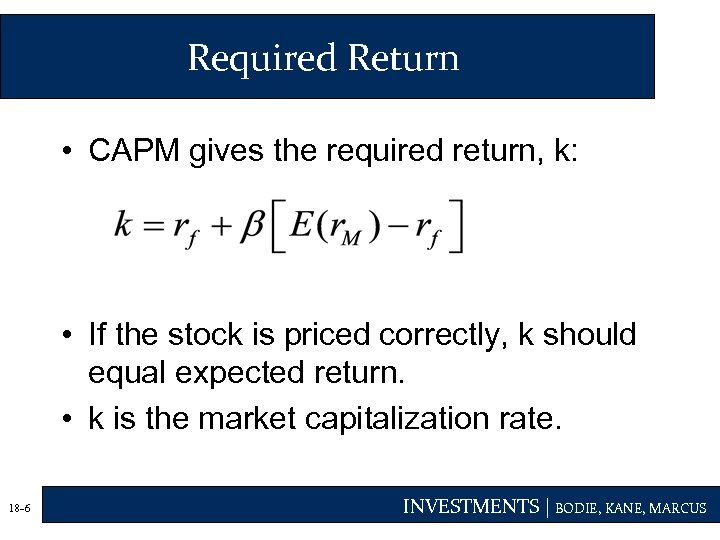Required Return • CAPM gives the required return, k: • If the stock is priced correctly, k should equal expected return. • k is the market capitalization rate. 18 -6 INVESTMENTS | BODIE, KANE, MARCUS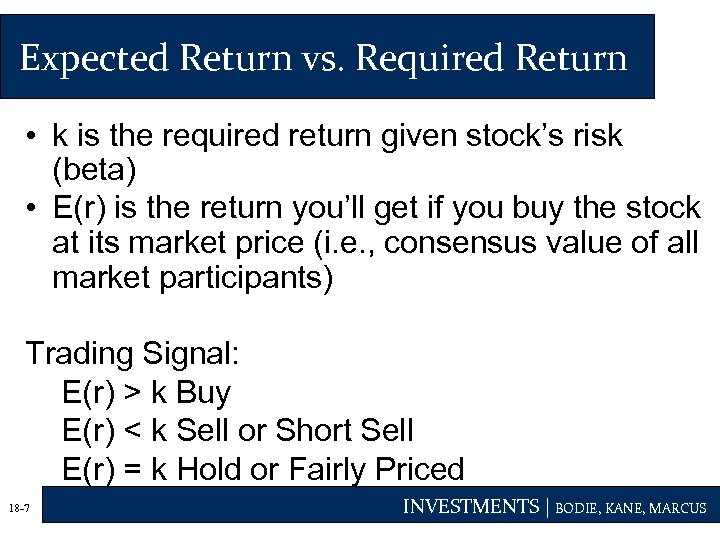Expected Return vs. Required Return • k is the required return given stock’s risk (beta) • E(r) is the return you’ll get if you buy the stock at its market price (i. e. , consensus value of all market participants) Trading Signal: E(r) > k Buy E(r) < k Sell or Short Sell E(r) = k Hold or Fairly Priced 18 -7 INVESTMENTS | BODIE, KANE, MARCUS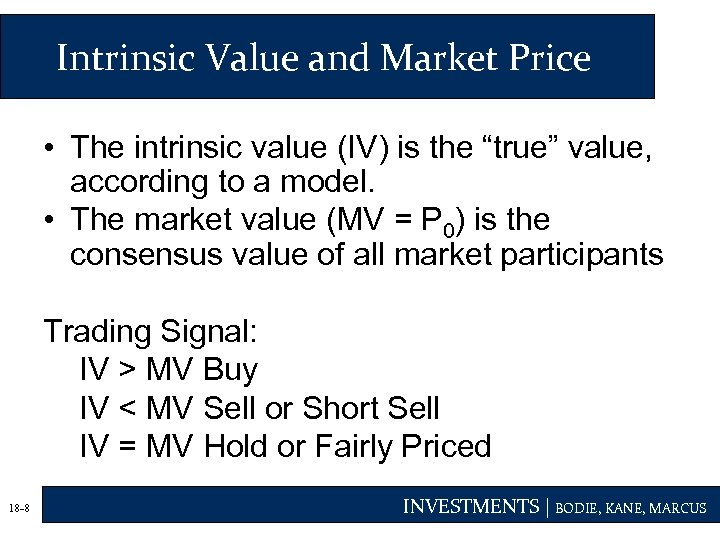Intrinsic Value and Market Price • The intrinsic value (IV) is the “true” value, according to a model. • The market value (MV = P 0) is the consensus value of all market participants Trading Signal: IV > MV Buy IV < MV Sell or Short Sell IV = MV Hold or Fairly Priced 18 -8 INVESTMENTS | BODIE, KANE, MARCUS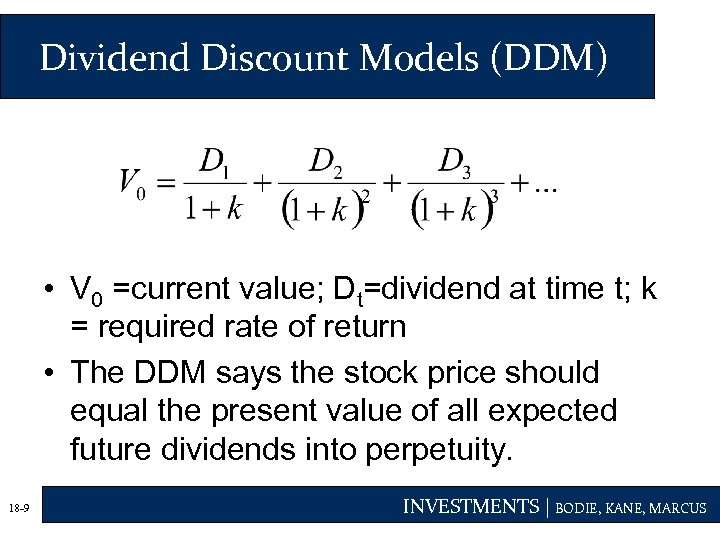Dividend Discount Models (DDM) • V 0 =current value; Dt=dividend at time t; k = required rate of return • The DDM says the stock price should equal the present value of all expected future dividends into perpetuity. 18 -9 INVESTMENTS | BODIE, KANE, MARCUS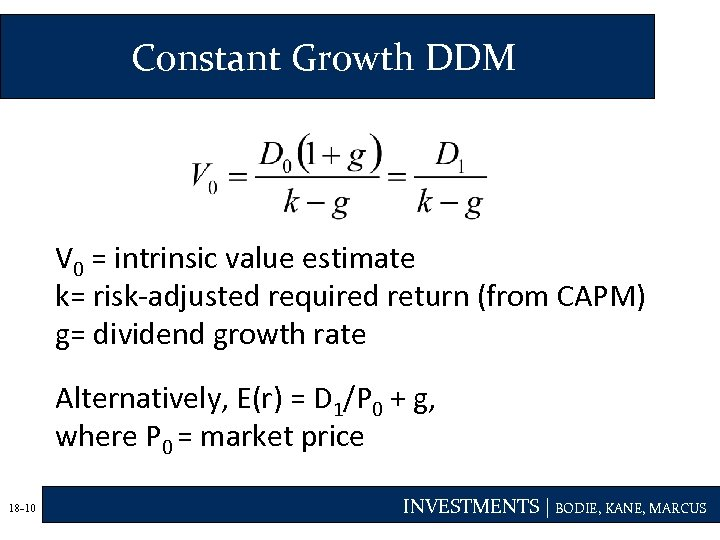Constant Growth DDM V 0 = intrinsic value estimate k= risk-adjusted required return (from CAPM) g= dividend growth rate Alternatively, E(r) = D 1/P 0 + g, where P 0 = market price 18 -10 INVESTMENTS | BODIE, KANE, MARCUS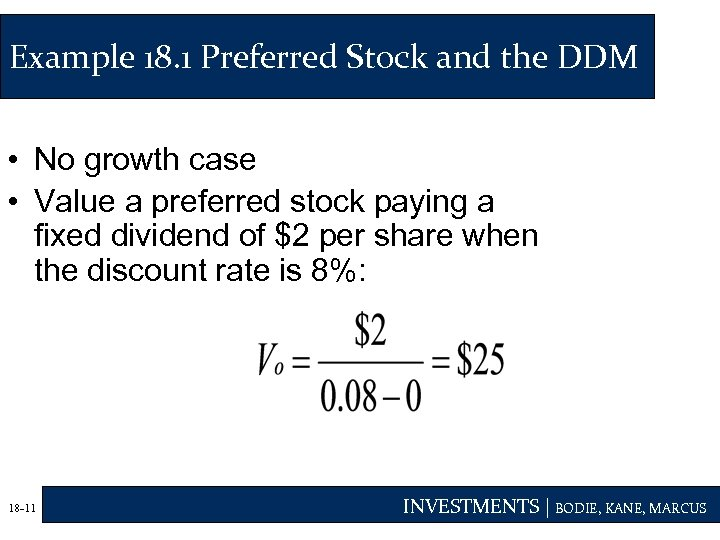Example 18. 1 Preferred Stock and the DDM • No growth case • Value a preferred stock paying a fixed dividend of \$2 per share when the discount rate is 8%: 18 -11 INVESTMENTS | BODIE, KANE, MARCUS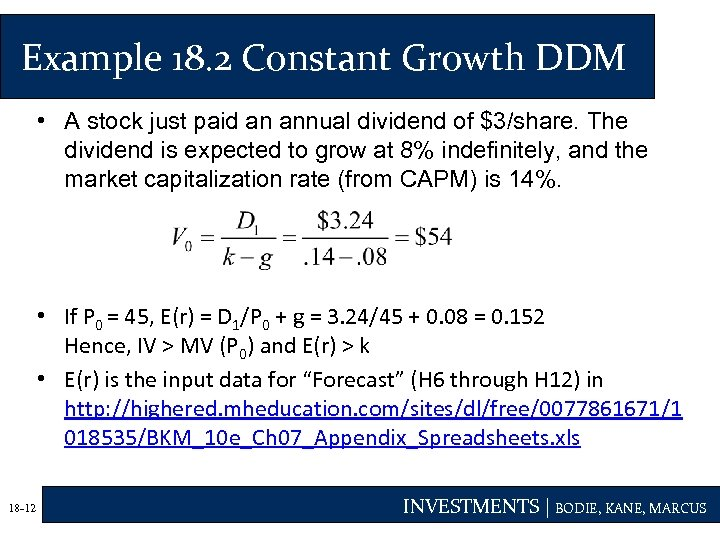Example 18. 2 Constant Growth DDM • A stock just paid an annual dividend of \$3/share. The dividend is expected to grow at 8% indefinitely, and the market capitalization rate (from CAPM) is 14%. • If P 0 = 45, E(r) = D 1/P 0 + g = 3. 24/45 + 0. 08 = 0. 152 Hence, IV > MV (P 0) and E(r) > k • E(r) is the input data for “Forecast” (H 6 through H 12) in http: //highered. mheducation. com/sites/dl/free/0077861671/1 018535/BKM_10 e_Ch 07_Appendix_Spreadsheets. xls 18 -12 INVESTMENTS | BODIE, KANE, MARCUS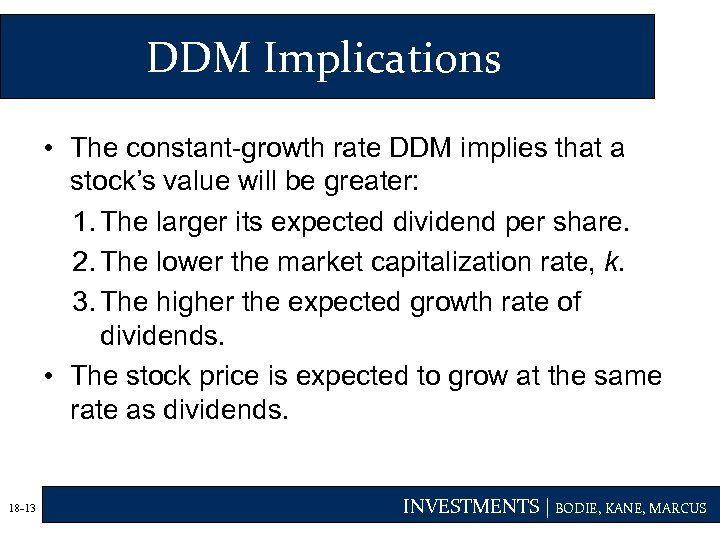DDM Implications • The constant-growth rate DDM implies that a stock’s value will be greater: 1. The larger its expected dividend per share. 2. The lower the market capitalization rate, k. 3. The higher the expected growth rate of dividends. • The stock price is expected to grow at the same rate as dividends. 18 -13 INVESTMENTS | BODIE, KANE, MARCUS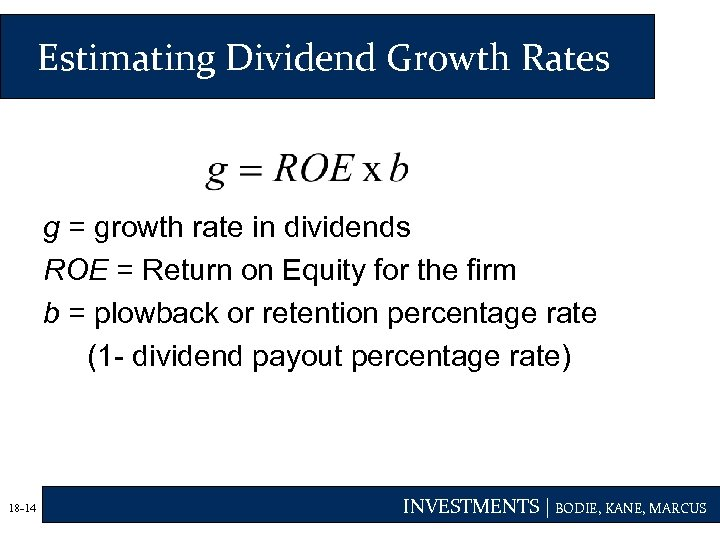Estimating Dividend Growth Rates g = growth rate in dividends ROE = Return on Equity for the firm b = plowback or retention percentage rate (1 - dividend payout percentage rate) 18 -14 INVESTMENTS | BODIE, KANE, MARCUS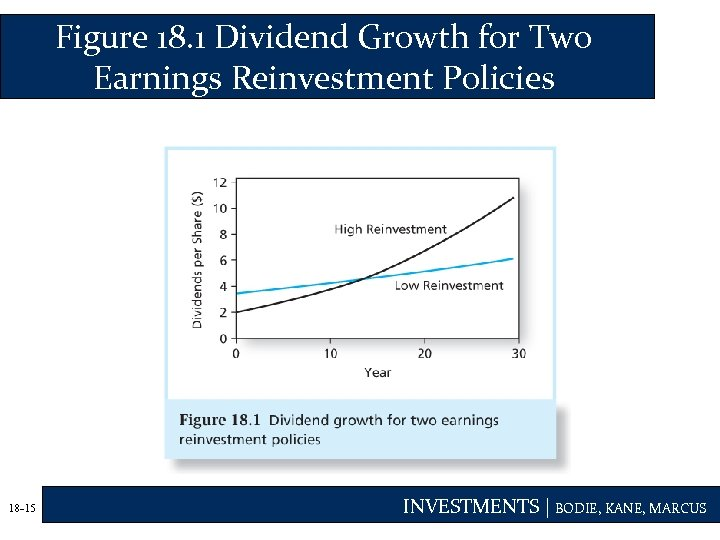Figure 18. 1 Dividend Growth for Two Earnings Reinvestment Policies 18 -15 INVESTMENTS | BODIE, KANE, MARCUS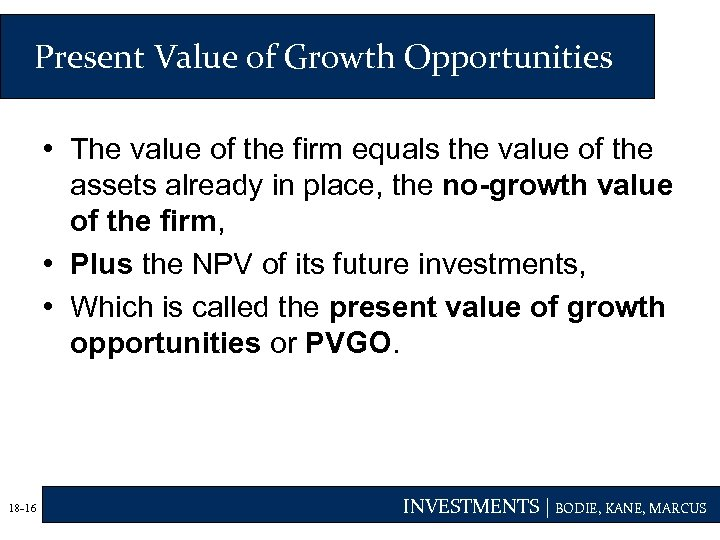Present Value of Growth Opportunities • The value of the firm equals the value of the assets already in place, the no-growth value of the firm, • Plus the NPV of its future investments, • Which is called the present value of growth opportunities or PVGO. 18 -16 INVESTMENTS | BODIE, KANE, MARCUS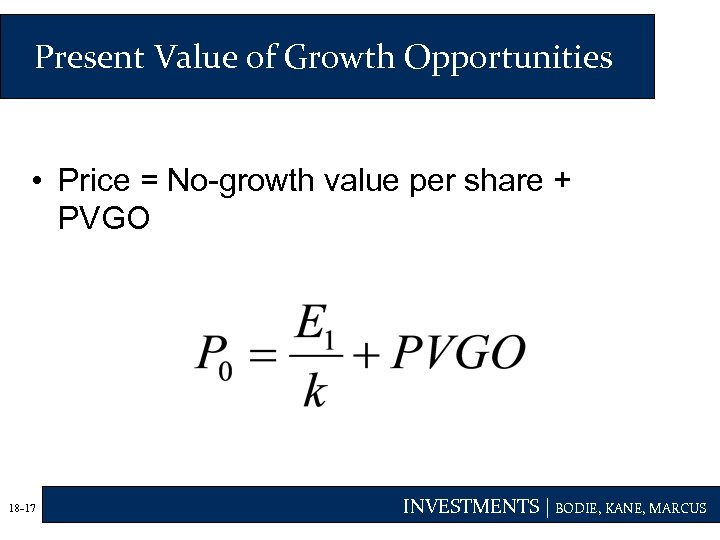Present Value of Growth Opportunities • Price = No-growth value per share + PVGO 18 -17 INVESTMENTS | BODIE, KANE, MARCUS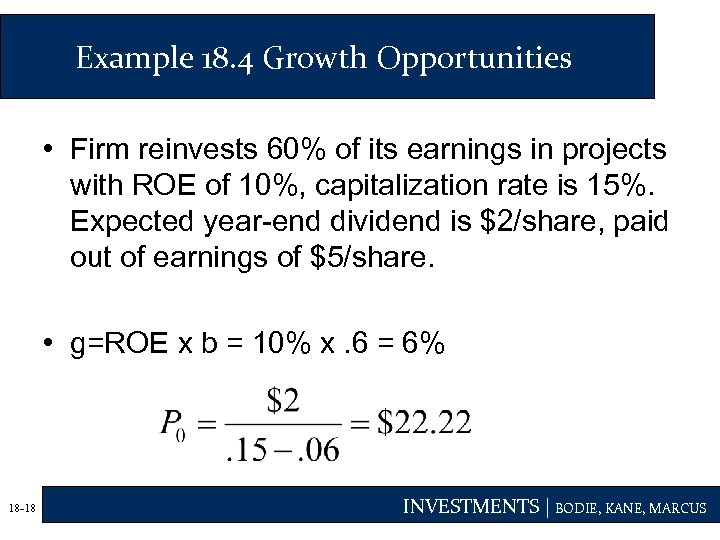Example 18. 4 Growth Opportunities • Firm reinvests 60% of its earnings in projects with ROE of 10%, capitalization rate is 15%. Expected year-end dividend is \$2/share, paid out of earnings of \$5/share. • g=ROE x b = 10% x. 6 = 6% 18 -18 INVESTMENTS | BODIE, KANE, MARCUS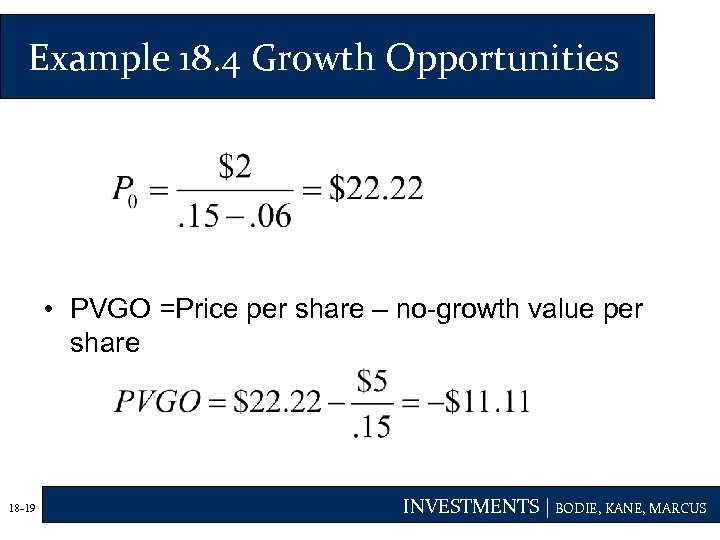Example 18. 4 Growth Opportunities • PVGO =Price per share – no-growth value per share 18 -19 INVESTMENTS | BODIE, KANE, MARCUS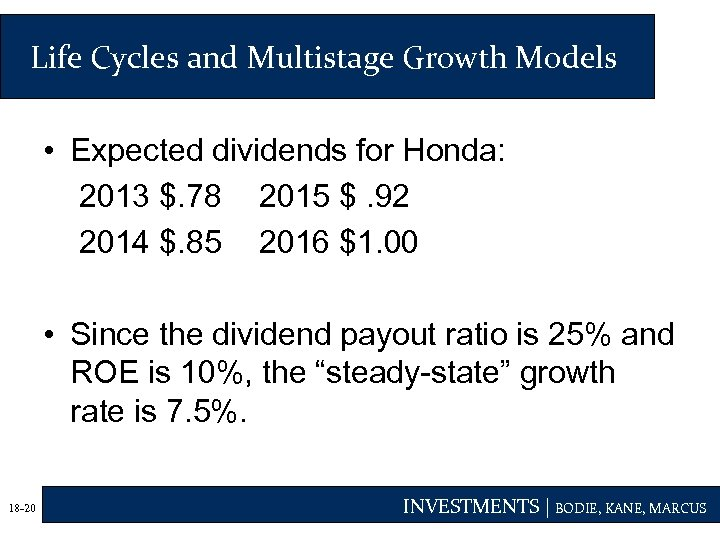Life Cycles and Multistage Growth Models • Expected dividends for Honda: 2013 \$. 78 2015 \$. 92 2014 \$. 85 2016 \$1. 00 • Since the dividend payout ratio is 25% and ROE is 10%, the “steady-state” growth rate is 7. 5%. 18 -20 INVESTMENTS | BODIE, KANE, MARCUS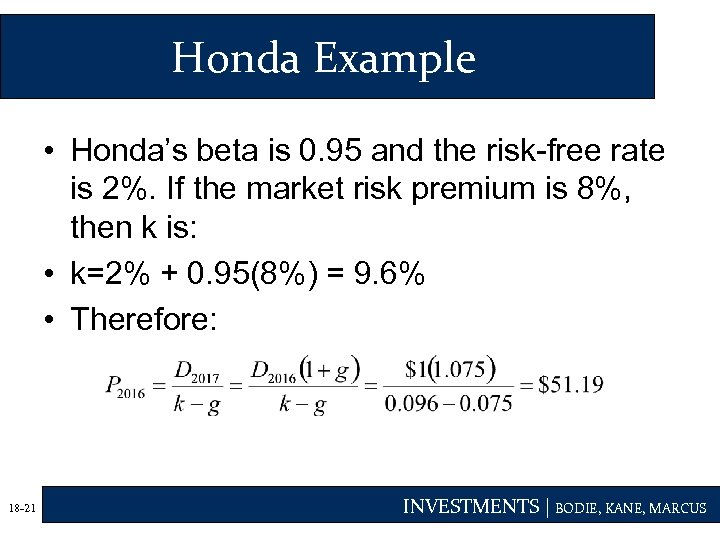Honda Example • Honda’s beta is 0. 95 and the risk-free rate is 2%. If the market risk premium is 8%, then k is: • k=2% + 0. 95(8%) = 9. 6% • Therefore: 18 -21 INVESTMENTS | BODIE, KANE, MARCUS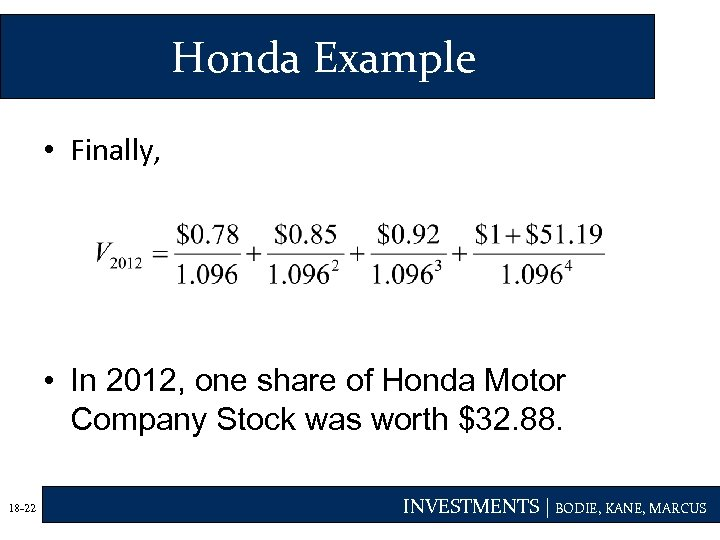Honda Example • Finally, • In 2012, one share of Honda Motor Company Stock was worth \$32. 88. 18 -22 INVESTMENTS | BODIE, KANE, MARCUS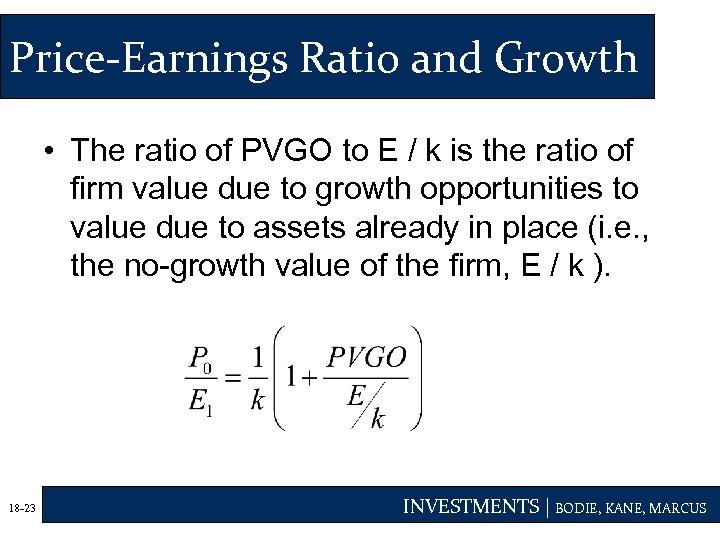Price-Earnings Ratio and Growth • The ratio of PVGO to E / k is the ratio of firm value due to growth opportunities to value due to assets already in place (i. e. , the no-growth value of the firm, E / k ). 18 -23 INVESTMENTS | BODIE, KANE, MARCUS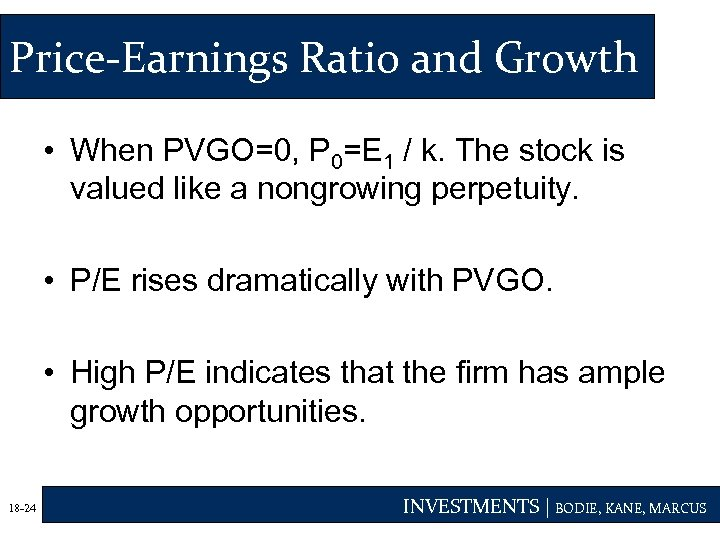Price-Earnings Ratio and Growth • When PVGO=0, P 0=E 1 / k. The stock is valued like a nongrowing perpetuity. • P/E rises dramatically with PVGO. • High P/E indicates that the firm has ample growth opportunities. 18 -24 INVESTMENTS | BODIE, KANE, MARCUS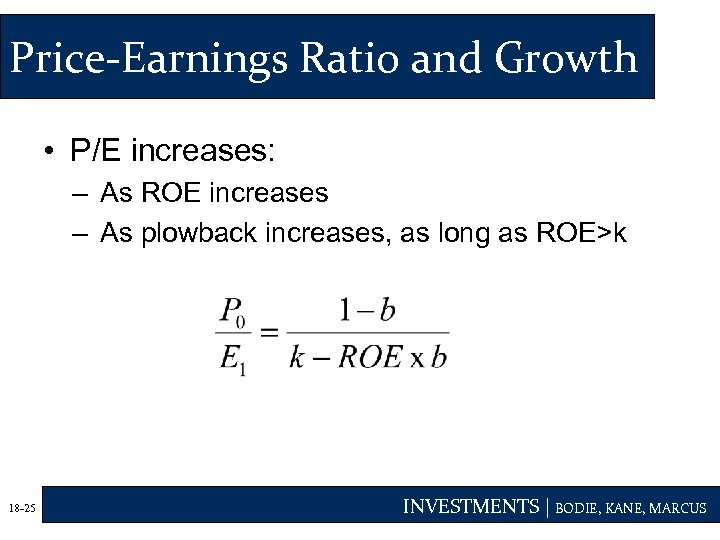Price-Earnings Ratio and Growth • P/E increases: – As ROE increases – As plowback increases, as long as ROE>k 18 -25 INVESTMENTS | BODIE, KANE, MARCUS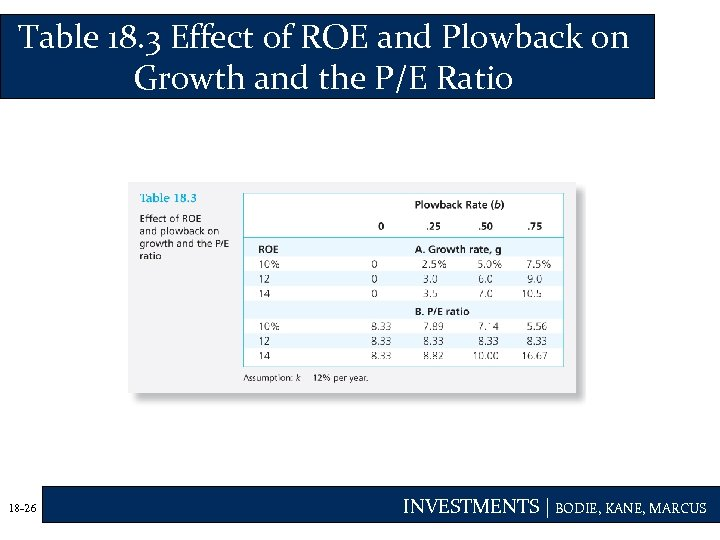Table 18. 3 Effect of ROE and Plowback on Growth and the P/E Ratio 18 -26 INVESTMENTS | BODIE, KANE, MARCUS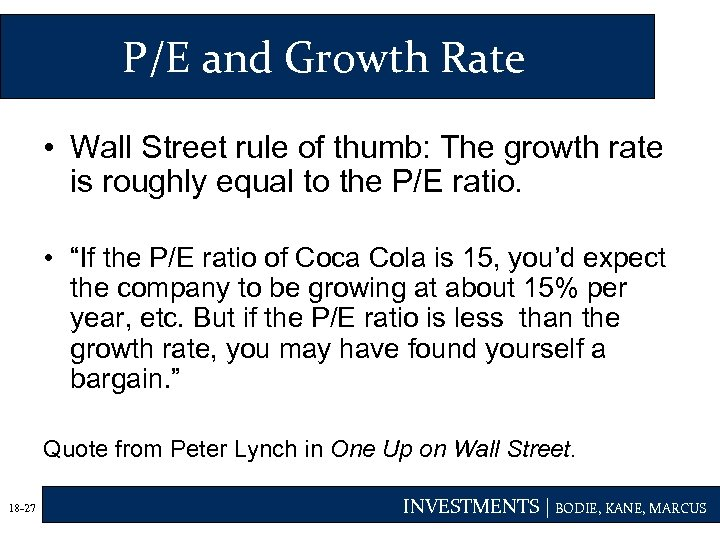P/E and Growth Rate • Wall Street rule of thumb: The growth rate is roughly equal to the P/E ratio. • “If the P/E ratio of Coca Cola is 15, you’d expect the company to be growing at about 15% per year, etc. But if the P/E ratio is less than the growth rate, you may have found yourself a bargain. ” Quote from Peter Lynch in One Up on Wall Street. 18 -27 INVESTMENTS | BODIE, KANE, MARCUS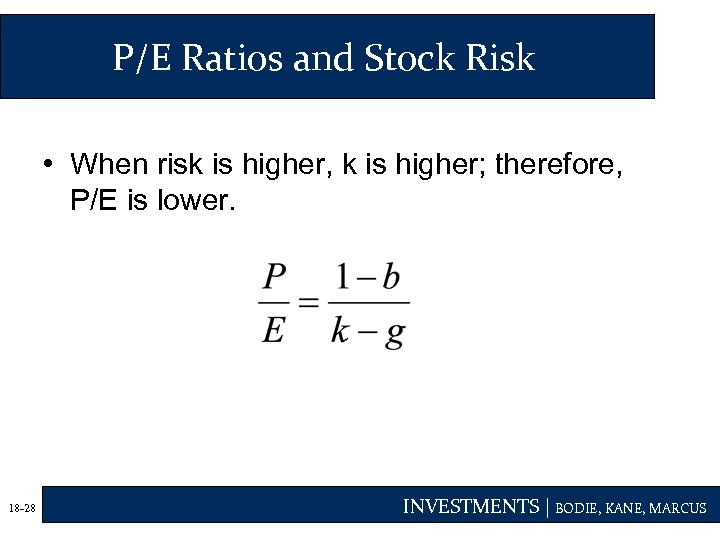P/E Ratios and Stock Risk • When risk is higher, k is higher; therefore, P/E is lower. 18 -28 INVESTMENTS | BODIE, KANE, MARCUS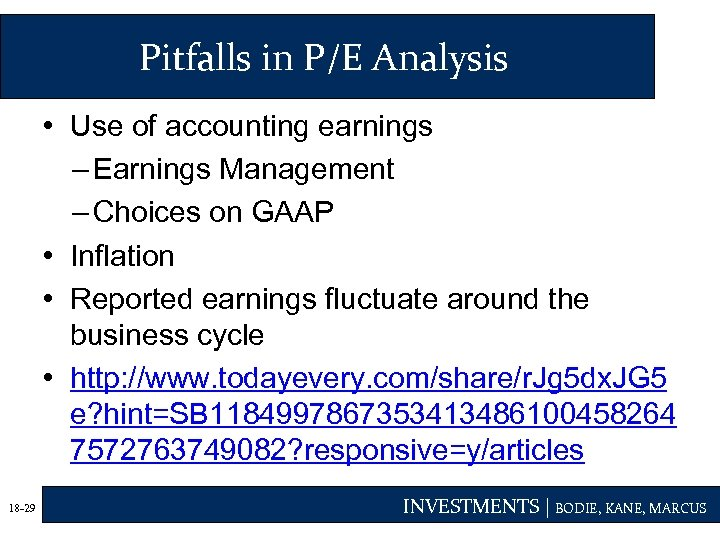Pitfalls in P/E Analysis • Use of accounting earnings – Earnings Management – Choices on GAAP • Inflation • Reported earnings fluctuate around the business cycle • http: //www. todayevery. com/share/r. Jg 5 dx. JG 5 e? hint=SB 1184997867353413486100458264 7572763749082? responsive=y/articles 18 -29 INVESTMENTS | BODIE, KANE, MARCUS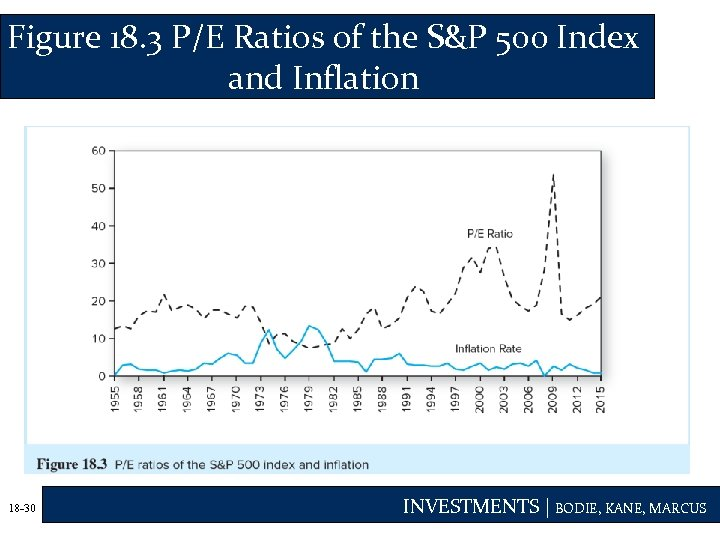Figure 18. 3 P/E Ratios of the S&P 500 Index and Inflation 18 -30 INVESTMENTS | BODIE, KANE, MARCUS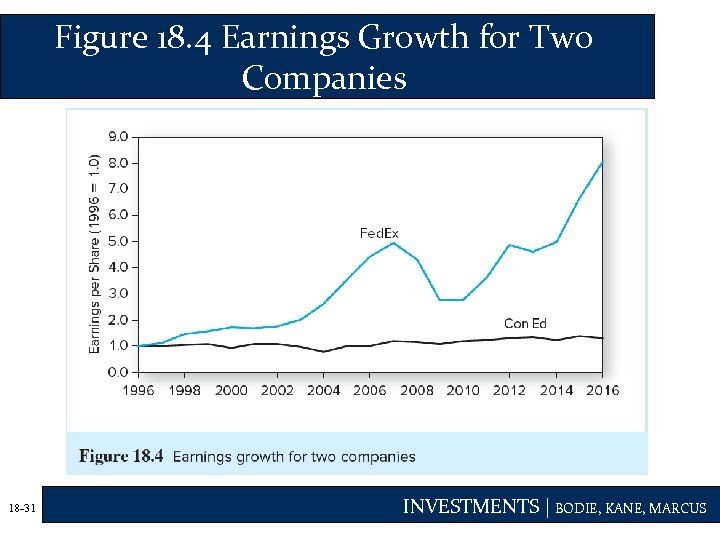Figure 18. 4 Earnings Growth for Two Companies 18 -31 INVESTMENTS | BODIE, KANE, MARCUS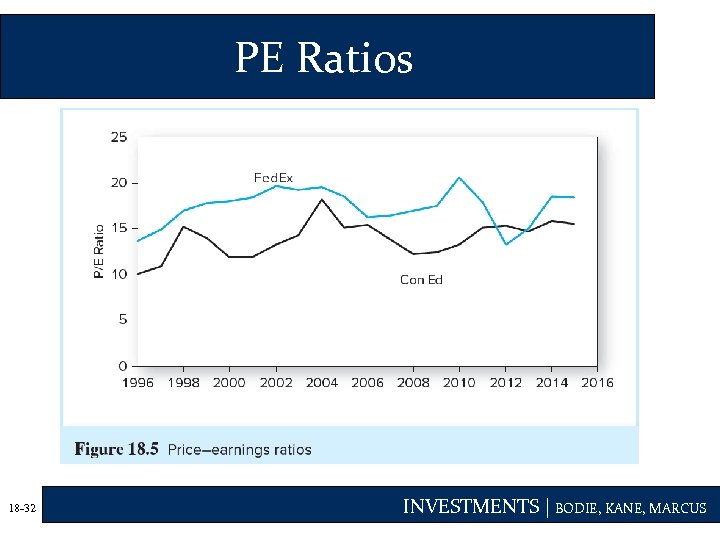PE Ratios 18 -32 INVESTMENTS | BODIE, KANE, MARCUS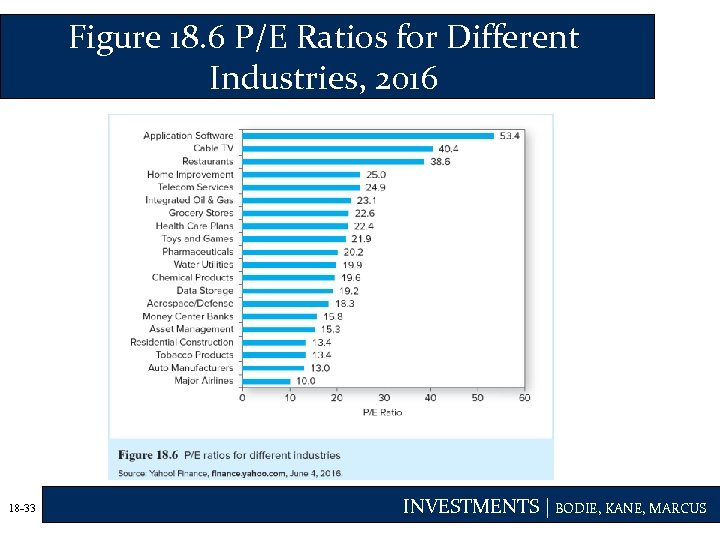Figure 18. 6 P/E Ratios for Different Industries, 2016 18 -33 INVESTMENTS | BODIE, KANE, MARCUS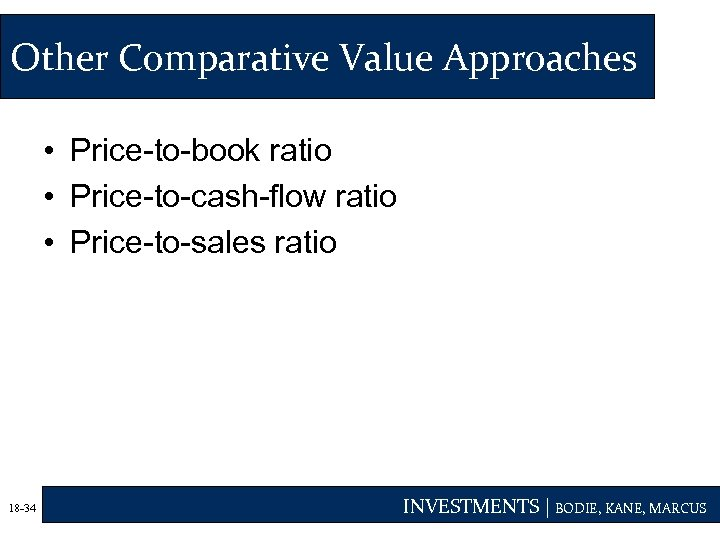Other Comparative Value Approaches • Price-to-book ratio • Price-to-cash-flow ratio • Price-to-sales ratio 18 -34 INVESTMENTS | BODIE, KANE, MARCUS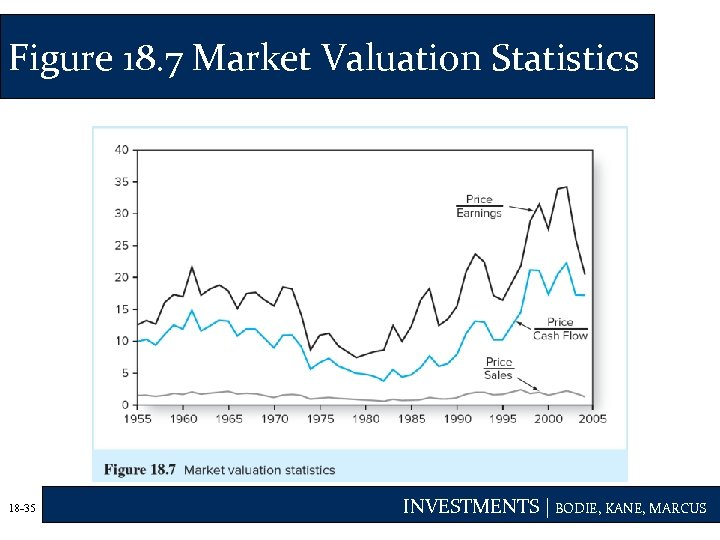Figure 18. 7 Market Valuation Statistics 18 -35 INVESTMENTS | BODIE, KANE, MARCUS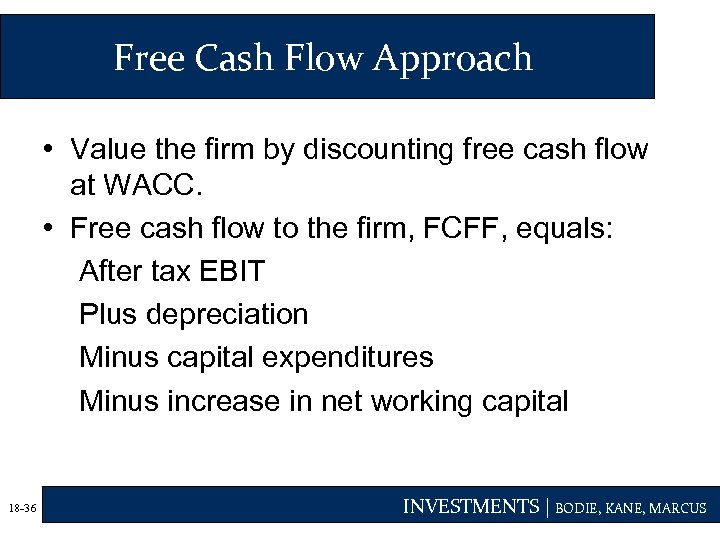Free Cash Flow Approach • Value the firm by discounting free cash flow at WACC. • Free cash flow to the firm, FCFF, equals: After tax EBIT Plus depreciation Minus capital expenditures Minus increase in net working capital 18 -36 INVESTMENTS | BODIE, KANE, MARCUS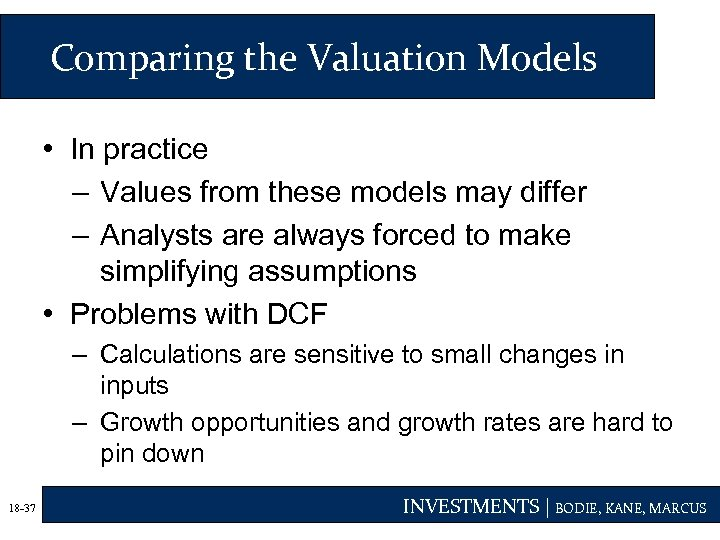Comparing the Valuation Models • In practice – Values from these models may differ – Analysts are always forced to make simplifying assumptions • Problems with DCF – Calculations are sensitive to small changes in inputs – Growth opportunities and growth rates are hard to pin down 18 -37 INVESTMENTS | BODIE, KANE, MARCUS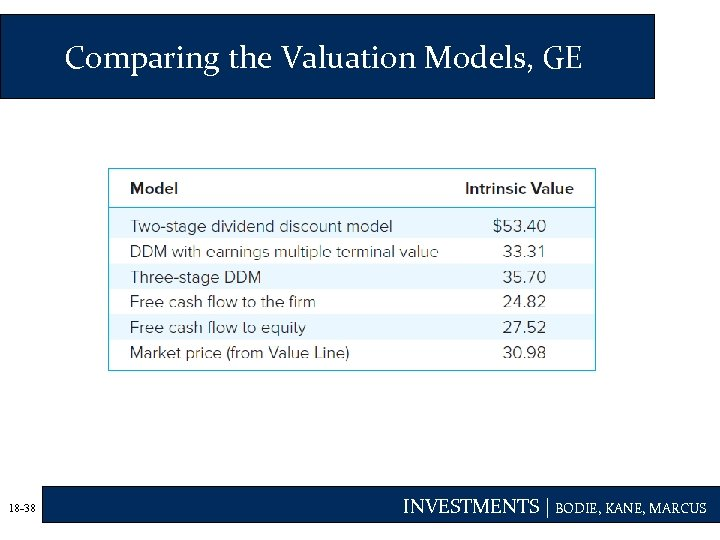Comparing the Valuation Models, GE 18 -38 INVESTMENTS | BODIE, KANE, MARCUS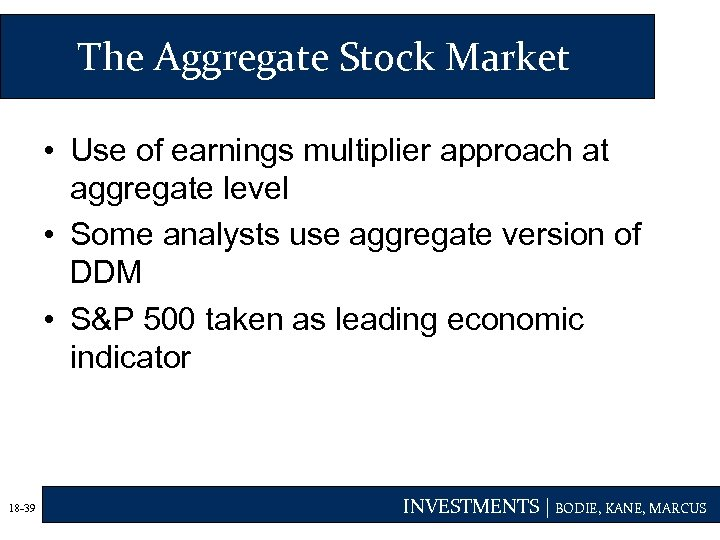The Aggregate Stock Market • Use of earnings multiplier approach at aggregate level • Some analysts use aggregate version of DDM • S&P 500 taken as leading economic indicator 18 -39 INVESTMENTS | BODIE, KANE, MARCUS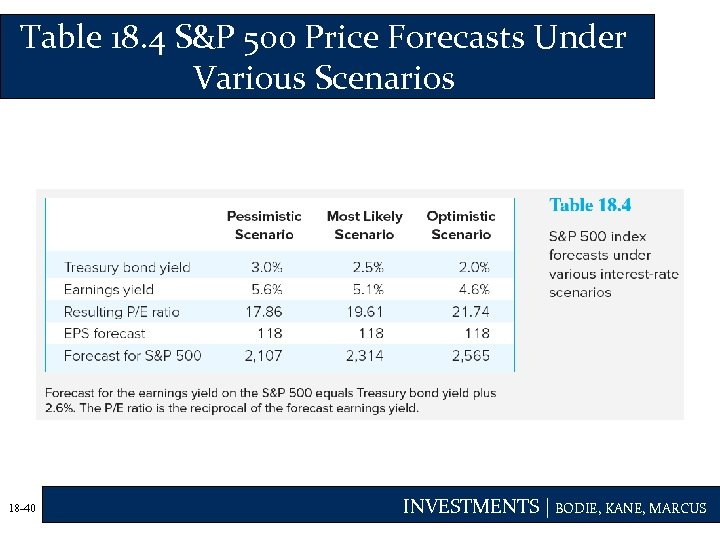Table 18. 4 S&P 500 Price Forecasts Under Various Scenarios 18 -40 INVESTMENTS | BODIE, KANE, MARCUS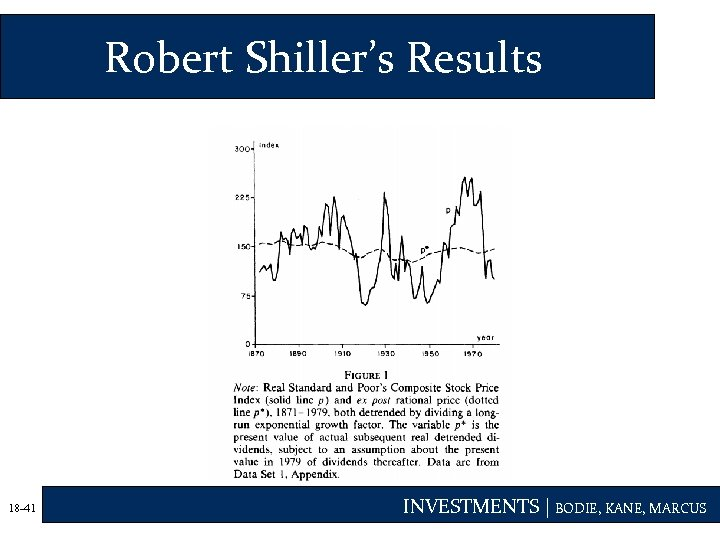Robert Shiller’s Results 18 -41 INVESTMENTS | BODIE, KANE, MARCUS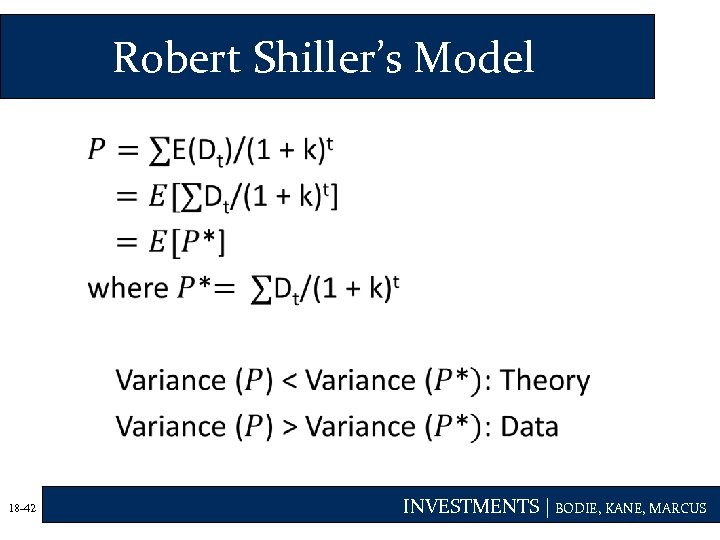Robert Shiller’s Model • 18 -42 INVESTMENTS | BODIE, KANE, MARCUS